# 为什么机器学习模型会失败？

• 2021 年 11 月 24 日
• 本文字数：4791 字

阅读完需：约 16 分钟## 模型训练

import pandas as pdpd.set_option('display.max_columns', None)train = pd.read_csv('train.csv', index_col='id')print(train)

Unnamed: 0 kjkrfgld bpowgknt raksnhjf vwpsxrgk  omtioxzz  yfmzwkruid                                                                          29252  2225    KfoTG    zPfZR    DtMvg      NaN      12.0      -3.0   98286  1598    ljBjd    THHLT    DtMvg    esAQH      21.0      -2.0   49040  7896    Lsuai    zPfZR    zeYAm    ZCIYy      12.0      -3.0   35261  1458    KfoTG    mDadf    zeYAm    ZCIYy      12.0      -1.0   98833  1817    KfoTG    THHLT    DtMvg    ARuYG      21.0      -4.0          tiwrsloh  weioazcf   poor  id                                29252      -1.0       0.5  False  98286      -5.0      -9.5   True  49040      -5.0      -9.5   True  35261      -5.0      -9.5  False  98833      -5.0      -9.5   True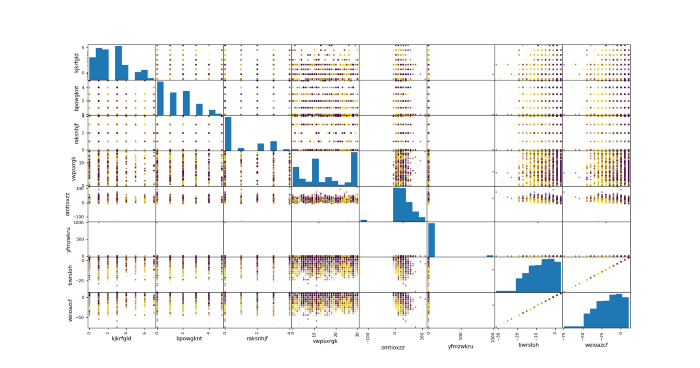## 支持向量机

from sklearn.pipeline import Pipelinefrom sklearn.preprocessing import RobustScalerfrom sklearn.neighbors import KNeighborsClassifiermodel = Pipeline(steps=preprocess+[                 ('scaler', RobustScaler()),                 ('estimator', KNeighborsClassifier(n_neighbors=5))])model.fit(X_train, y_train)y_pred = model.predict(X_test)print(classification_report(y_test,y_pred))

precision    recall  f1-score   support       False       0.73      0.77      0.75       891        True       0.70      0.66      0.68       750    accuracy                           0.72      1641   macro avg       0.72      0.71      0.71      1641weighted avg       0.72      0.72      0.72      1641

## KNN

from sklearn.pipeline import Pipelinefrom sklearn.preprocessing import RobustScalerfrom sklearn.neighbors import KNeighborsClassifiermodel = Pipeline(steps=preprocess+[                 ('scaler', RobustScaler()),                 ('estimator', KNeighborsClassifier(n_neighbors=5))])model.fit(X_train, y_train)y_pred = model.predict(X_test)print(classification_report(y_test,y_pred))

precision    recall  f1-score   support       False       0.71      0.74      0.72       891        True       0.67      0.63      0.65       750    accuracy                           0.69      1641   macro avg       0.69      0.69      0.69      1641weighted avg       0.69      0.69      0.69      1641

## 梯度提升

from sklearn.pipeline import Pipelinefrom sklearn.ensemble import GradientBoostingClassifiermodel = Pipeline(steps=preprocess+[                 ('estimator',                   GradientBoostingClassifier(max_depth=5,                                             n_estimators=100))])model.fit(X_train, y_train)y_pred = model.predict(X_test)print(classification_report(y_test,y_pred))

precision    recall  f1-score   support       False       0.76      0.78      0.77       891        True       0.73      0.70      0.72       750    accuracy                           0.74      1641   macro avg       0.74      0.74      0.74      1641weighted avg       0.74      0.74      0.74      1641

## 模型预测分布

import matplotlib.pyplot as pltpd.DataFrame(model.predict_proba(X_train)).hist()plt.show()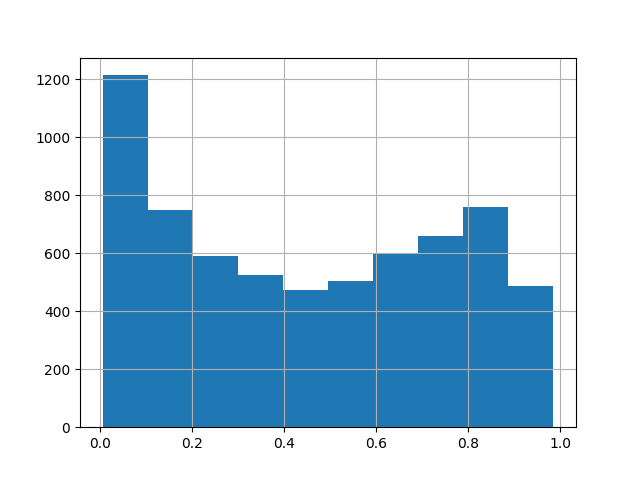pd.DataFrame(model.predict_proba(X_test)).hist()plt.show()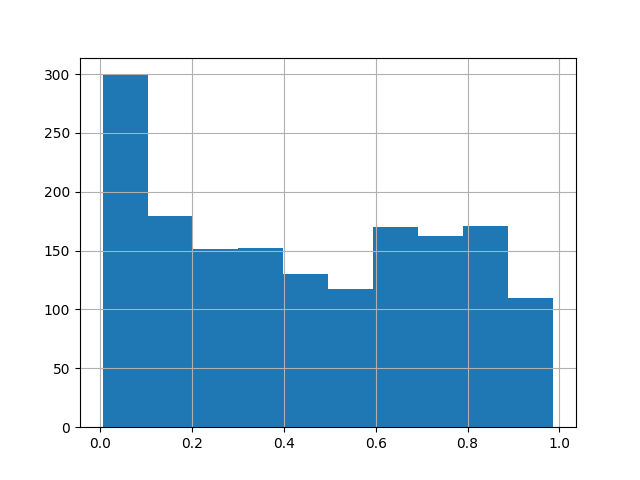## 偏差分布

train_proba = model.predict_proba(X_train)[:,1]pd.DataFrame(train_proba-y_train.astype(int)).hist(bins=50)plt.show()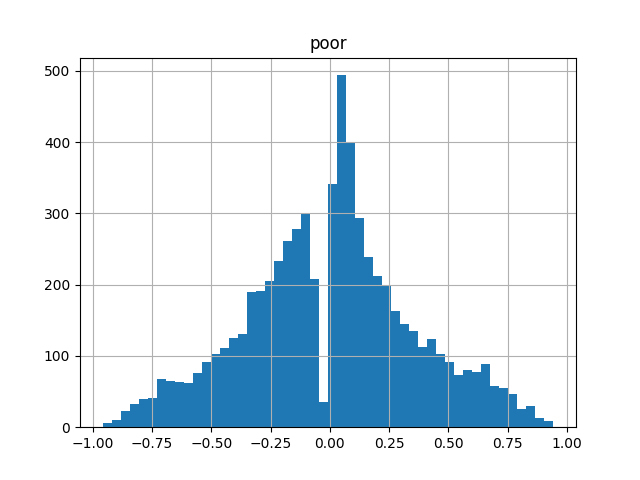test_proba = model.predict_proba(X_test)[:,1]pd.DataFrame(test_proba-y_test.astype(int)).hist(bins=50)plt.show()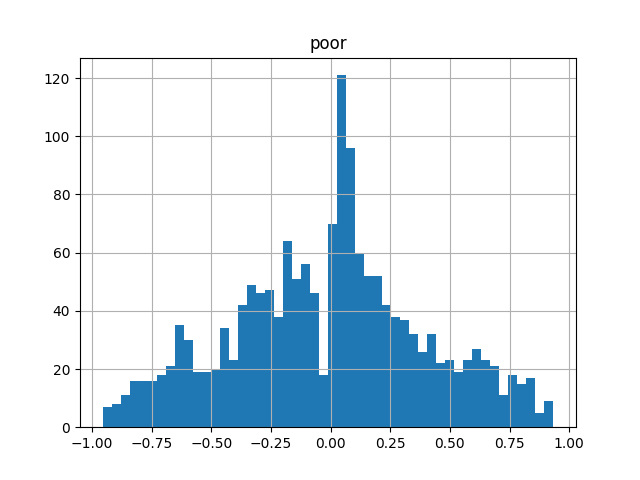## 验证正态性

import scipyscipy.stats.normaltest(train_proba-y_train.astype(int))

NormaltestResult(statistic=15.602215177113427, pvalue=0.00040928141243470884)

## 模型可解释性

cols = X_train.columnsvals= dict(model.steps)['estimator'].feature_importances_plt.figure()plt.bar(cols, vals)plt.show()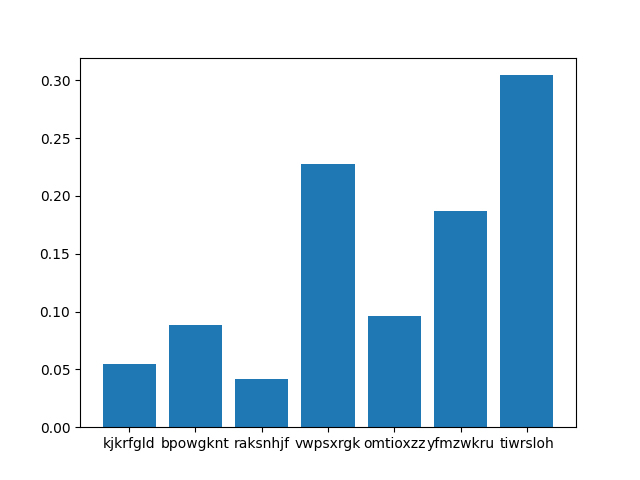zero_mask = model.predict_proba(X_train)[:,1]<=0.1one_mask = y_train==1mask = np.logical_or(zero_mask,one_mask)X_train = X_train.loc[mask,:]y_train = y_train.loc[mask]model.fit(X_train,y_train)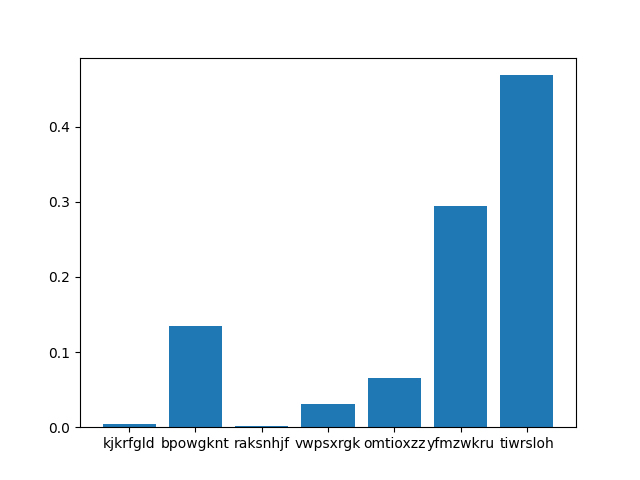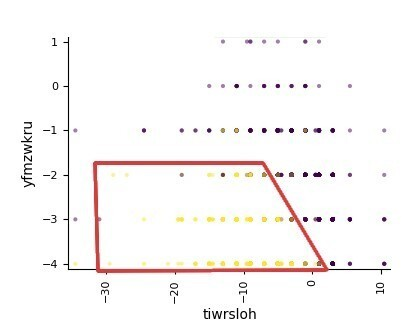## 我们学到了什么？

1. 尝试不同的函数族模型和超参数，并确认所有的下降在性能上处于平稳状态。

2. 为得到最好的解释，计算模型的输出分布和目标的偏差分布。如果数据是问题所在，则输出必须是均匀的，并且偏差必须遵循高斯分布。

3. 尽管数据是问题所在，但试着从模型输出和偏差分布中找到一个模型表现良好的区域。尝试对这个区域进行分割和定性，例如，用这个子集重新训练模型，并提取其可解释性。

4. 此外，在表征某些子集时，我们可以尝试从业务知识中思考，问题是来自数据的统计/测量噪音，还是来自缺乏预测因变量所需的一些特征值。

2021 年 11 月 24 日 10:003## 评论

• ###### 云端数智新引擎，腾讯云原生数据湖计算重磅发布

腾讯云发布云原生数据湖计算服务，协同腾讯云端数据湖体系，帮助企业高效构建云端数据湖架构、降低数据计算成本，提升数据分析敏捷性、激发数据应用价值，助力企业数字化决策。

这周，又有大事儿了。

越玩越上头

• ###### 是 AI 大神也是创业狂人！继收获首个 IPO 后，吴恩达旗下公司融资 5700 万美元

虽然当前市场上充斥着大量人工智能带来变革的言论，但吴恩达坦言，他在工厂看到的情况是，人工智能并没有得到广泛应用。

• ###### 官宣！达摩院开源秘藏深度语言模型体系 AliceMind，NLP 正在走向大工业时代

AliceMind 开源将降低 NLP 领域研究和应用创新的门槛，助推行业从手工业时代走向大工业时代

• ###### 如何高效实现图片搜索？Dropbox 的核心方法和架构优化实践

在这篇文章中，我们将基于机器学习中的技术描述图像内容搜索方法背后的核心思想，然后讨论如何在Dropbox现有的搜索基础架构上构建高效的实现。

• ###### 从感知智能迈向认知智能，百分点科技找到了一条跑得通的 AI 落地新范式

百分点团队发现，基于符号主义做引导，再结合连接主义和行为主义的方法，是比较好的能在行业里落地，且能够真正产生应用价值的方案。

AI技术的攻与防

• ###### 英伟达 CEO 黄仁勋对收购 Arm 信心满满，欧盟却表示十分担忧

威胁主要来自于这项交易对欧盟“技术主权”理想的挑战。个欧盟国家将向欧洲芯片行业注资数百亿欧元的“欧洲共同利益重要计划”。

7月日更

rust

#### 懂了！时间复杂度O(1)，O(logn) ，O(n)，O(nlogn)...